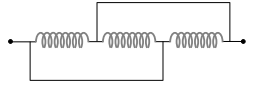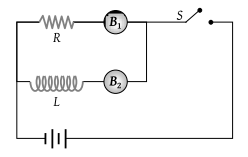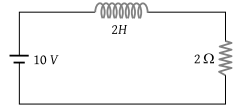The self inductance of a coil is L. Keeping the length and area same, the number of turns in the coil is increased to four times. The self inductance of the coil will now be

(1) $\frac{1}{4}L$

(2) L

(3) 4 L

(4) 16 L

Concept Questions :-

Self-inductance
High Yielding Test Series + Question Bank - NEET 2020

Difficulty Level:

A coil has an inductance of 2.5 H and a resistance of 0.5 r. If the coil is suddenly connected across a 6.0 volt battery, then the time required for the current to rise 0.63 of its final value is

(1) 3.5 sec

(2) 4.0 sec

(3) 4.5 sec

(4) 5.0 sec

Concept Questions :-

LR circuit
High Yielding Test Series + Question Bank - NEET 2020

Difficulty Level:

Pure inductance of 3.0 H is connected as shown below. The equivalent inductance of the circuit is(1) 1 H

(2) 2 H

(3) 3 H

(4) 9 H

Concept Questions :-

Self-inductance
High Yielding Test Series + Question Bank - NEET 2020

Difficulty Level:

If a current of 10 A flows in one second through a coil, and the induced e.m.f. is 10 V, then the self-inductance of the coil is

(1) $\frac{2}{5}H$

(2) $\frac{4}{5}H$

(3) $\frac{5}{4}H$

(4) 1 H

Concept Questions :-

Self-inductance
High Yielding Test Series + Question Bank - NEET 2020

Difficulty Level:

The adjoining figure shows two bulbs B1 and B2 resistor R and an inductor L. When the switch S is turned off(1) Both B1 and B2 die out promptly

(2) Both B1 and B2 die out with some delay

(3) B1 dies out promptly but B2 with some delay

(4) B2 dies out promptly but B1 with some delay

Concept Questions :-

Self-inductance
High Yielding Test Series + Question Bank - NEET 2020

Difficulty Level:

An inductance L and a resistance R are first connected to a battery. After some time the battery is disconnected but L and R remain connected in a closed circuit. Then the current reduces to 37% of its initial value in

(1) RL sec

(2) $\frac{R}{L}sec$

(3) $\frac{L}{R}sec$

(4) $\frac{1}{LR}sec$

Concept Questions :-

LR circuit
High Yielding Test Series + Question Bank - NEET 2020

Difficulty Level:

In an LR-circuit, the time constant is that time in which current grows from zero to the value (where I0 is the steady-state current)

(1) 0.63 I0

(2) 0.50 I0

(3) 0.37 I0

(4) I0

Concept Questions :-

LR circuit
High Yielding Test Series + Question Bank - NEET 2020

Difficulty Level:

In the figure magnetic energy stored in the coil is(1) Zero

(2) Infinite

(3) 25 joules

(4) None of the above

Concept Questions :-

Self-inductance
High Yielding Test Series + Question Bank - NEET 2020

Difficulty Level:

The core of a transformer is laminated to reduce energy losses due to

(1) Eddy currents

(2) Hysteresis

(3) Resistance in winding

(4) None of these

Concept Questions :-

Eddy current
High Yielding Test Series + Question Bank - NEET 2020

Difficulty Level:

When the speed of a dc motor increases the armature current

(1) Increases

(2) Decreases

(3) Does not change

(4) Increases and decreases continuously

Concept Questions :-

Motional emf